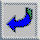# Practical numbers

A positive integer m is said to be practical if every n with 1nm is a sum of distinct divisors of m.
Some article about practical numbers can be found on line in my papers. P. Erdos, W. Sierpinski, G. Tenenbaum, E. Saias B.M. Stewart, H.N. Shapiro, M. Margenstern, M. Hausman, and others dealt with practical numbers in their papers.
Table of practical numbers
Table of twin practical numbers
Table of triplets of practical numbers
Table of 5-uples of practical numbers

(Giuseppe Melfi - Giuseppe.Melfi@unine.ch)Giuseppe Melfi's home page